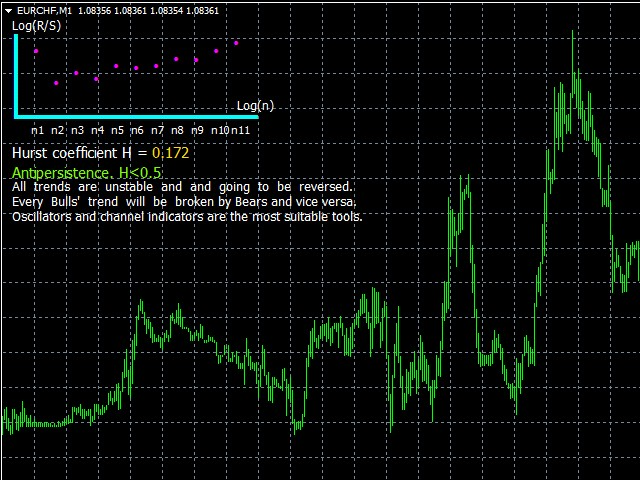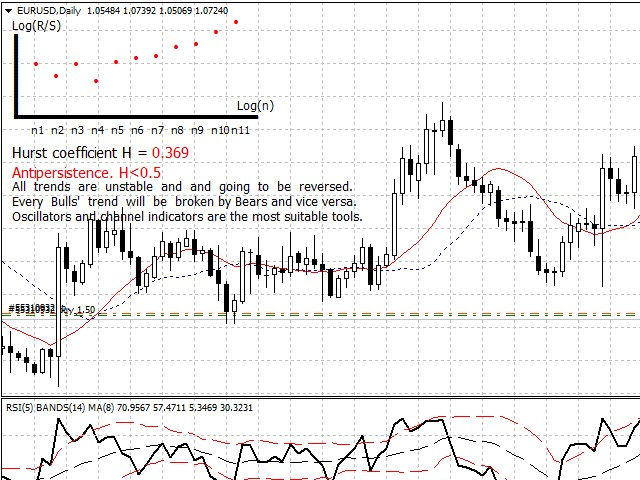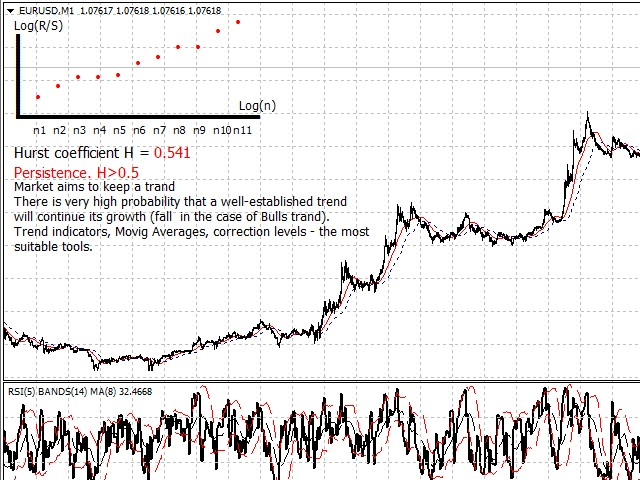# Hurst Index

\$12.99 \$35.00

-63%\$12.99 \$35.00

# Hurst Index

“Hurst index” is the analyzer of the the selected currency pair market fractal structure by using the R/S-analysis.

The analyzer determines, by calculating Hurst index, whether the market tends to keep the trend (persistence), or direction in the past most likely will be replaced by the opposite direction in the future (antipersistence).

### Hurst Exponent

The Hurst exponent is used as a measure of long-term memory of time series. Studies involving the Hurst exponent were originally developed in hydrology for the practical matter of determining optimum dam sizing for the Nile river‘s volatile rain and drought conditions that had been observed over a long period of time.  Benoît Mandelbrot believes the same effect can be generalized on Financial markets.

• If the Hurst index ranges from 0.5 to 1the process must be characterized by long-term memory. In other words, there is persistence.
For example, “blue chips” (Apple, IBM, Microsoft stocks) have persistent time serieses.)
• If the Hurst index ranges from 0 to 0.5, the process must be characterized by antipersistence. For example, second-tier stocks.

### Analyzer principle of calculation

1. The analyzer calculates Hurst index for the last 1000 or 100 bars;
2. The graph, numeric value of Hurst index and conclusion will be shown on the main window;
3. If new bar have formed analizer recalculate coefficient.

### Input parameters

1. NumberOfBars – Set the number of investigated bars. The key parameter. Hurst index can be calculated for 1000 or 100 bars. If you try to specify a different value an error message will appear on the screen. For the purity of the experiment it is highly recommended to use 1000 bars for calculation that ensure the accuracy, significance and reliability of calculations;
2. OffsetX – set the ofset of the analyzer graph on the program main window relative to the horizontal axis. Remember: the “zero point” is the upper-left corner of the window.
3. OffsetY – set the ofset of the analyzer graph on the program main window relative to the vertical axis.
4. SetAxisXcolor – X-axis color.
5. SetAxisYcolor – Y-axis color.
6. SetTextColor -Text color.
7. SetHurstColor – numeric value of Hurst index color.
8. SetGraphColor – the graph points color.

(!) It is highly recommended to use default values.

### How to use